# Variations 4/2

Determine the number of items when the count of variations of fourth class without repeating is 600 times larger than the count of variations of second class without repetition.

n =  27

### Step-by-step explanation: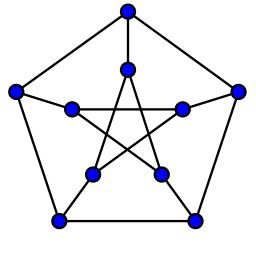Did you find an error or inaccuracy? Feel free to write us. Thank you!Tips to related online calculators
Looking for help with calculating roots of a quadratic equation?
Would you like to compute count of combinations?

## Related math problems and questions:

• VariationsDetermine the number of items when the count of variations of fourth class without repeating is 42 times larger than the count of variations of third class without repetition.
• Variation equation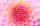Solve combinatorics equation: V(2, x+8)=72 . .. variations second class from x+8 items
• Variations 3rd classFrom how many elements we can create 13,800 variations 3rd class without repeating?
• CombinationsFrom how many elements we can create 990 combinations 2nd class without repeating?
• CombinationsHow many elements can form six times more combinations fourth class than combination of the second class?
• 2nd class variationsFrom how many elements you can create 2450 variations of the second class?
• First class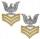The shipment contains 40 items. 36 are first grade, 4 are defective. How many ways can select 5 items, so that it is no more than one defective?
• CombinationsIf the number of elements increase by 3, it increases the number of combinations of the second class of these elements 5 times. How many are the elements?
• VCP equationSolve the following equation with variations, combinations and permutations: 4 V(2,x)-3 C(2,x+ 1) - x P(2) = 0
• STRESSED wordEach letter in STRESSED is printed on identical cards, one letter per card, and assembled in random order. Calculate the probability that the cards spell DESSERTS when assembled.
• 2nd class combinationsFrom how many elements you can create 4560 combinations of the second class?
• Party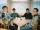At the party everyone clink with everyone. Together, they clink 406 times. How many people were at the party?
• Points in space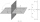There are n points, of which no three lie on one line and no four lies on one plane. How many planes can be guided by these points? How many planes are there if there are five times more than the given points?
• SalamiHow many ways can we choose 5 pcs of salami if we have 6 types of salami for 10 pieces and one type for 4 pieces?
• PIN codePIN on Michael credit card is a four-digit number. Michael told this to his friend: • It is a prime number - that is, a number greater than 1, which is only divisible by number one and by itself. • The first digit is larger than the second. • The second d
• Jane classWhen asked how many students are in class, Jane said, if we increase the number of students in our class by a hundred % and then add half the number of students, we get 100. How many students are in Jane's class?
• Five-digit numbersHow many different five-digit numbers can be created from the numbers 2,3,5 if the number 2 appears in the number twice and the number 5 also twice?